## 10 Apr 2016

### Frege (§7) Begriffsschrift, Chapter 1 (Geach transl.), "Negation", summary

[Search Blog Here. Index-tags are found on the bottom of the left column.]

[The following is summary. Bracketed commentary is my own. Please forgive my typos, as proofreading is incomplete.]

Summary of

Gottlob Frege

Begriffsschrift, Chapter 1
(Geach transl.)

§7 Negation

Brief Summary:
We use a small vertical stroke to mean negation in Frege’s symbolic system. This means the negation of A.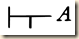We can then symbolize all operators using just negation and the conditional.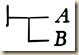means BA.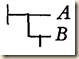means ¬B→A, and this expresses the inclusive disjunctive ‘or”.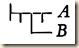means ¬(B→¬A), and this is equivalent to the conjunctive “and”.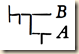means ¬(¬A→B), and it has the meaning of “neither ... nor ...”.

Summary

Frege has been describing his logic notation system. [Let us first review some terms and parts of the symbolization. In his symbols, there are letters and horizontal lines. If there are just those two components, then they just signify an idea, but not yet the sorts of judgments that we deal with in logic. So if we just havethis might only, for example, “produce in the reader the idea of the mutual attraction of unlike magnetic poles.” (We might also make inferences from this idea, in which case we would see it as saying “the circumstances that unlike magnetic poles attract one another” or “the proposition that unlike magnetic poles attract one another”. See section 2.) This horizontal line is the content stroke, and it “combines the symbols following it into a whole”.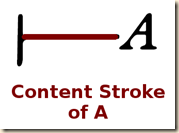At the left side we can place a vertical stroke,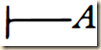which is the judgment stroke of A.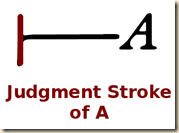This turns the idea into a judgment (or perhaps we can think of it also as a proposition that asserts a judgment). So in our example, our judgment could be: “unlike magnetic poles attract one another” (1).]

He now will explain how to mark negations. He writes:

If a small vertical stroke is attached to the lower side of the content-stroke, this shall express the circumstance of the content's not being the case.
(7)

So consider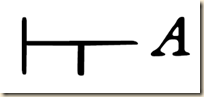This means: “A is not the case”. [In our example from above, this might be: “it is not the case that unlike magnetic poles attract one another”.] “I call this small vertical stroke the negation-stroke” (7).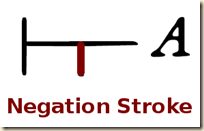“The part of the horizontal stroke occurring to the right of the negation-stroke is the content-stroke of A” (7d).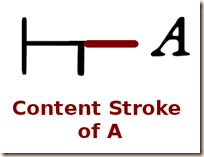“the part occurring | to the left of the negation-stroke is the content-stroke of the negation of A” (7-8).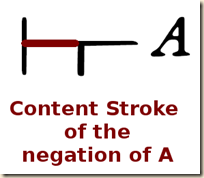But if there is no judgment stroke to the negation,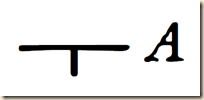then this “merely requires the formation of the idea that A is not the case, without expressing whether this idea is true” (8). [Recall from our example before with the simple affirmative form, that when there was no judgment stroke, it would merely “produce in the reader the idea of the mutual attraction of unlike magnetic poles.” So perhaps in this case it produces in the reader the idea that unlike magnetic poles do not attract one another.]

[Recall that in Frege’s system, in the conditional form: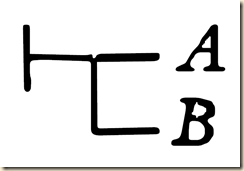B is the antecedent and A is the consequent (even though we might expect it to be the other way around, in alphabetical order).] Frege now will examine how the negation can be applied in the conditional forms. [I will need to make a guess here, but I will explain. I am going to guess that for Frege, the overall formulation for the conditional, where A is the consequent and B is the antecedent, would be: “The case where B is to be affirmed and A is to be denied does not occur”. This of course is because this is the only instance where the whole conditional is false. I say this, because see how he explains the negation of the consequent.]

We now deal with some cases where the symbols of conditionality and negation are combined.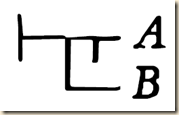means: ‘the case in which B is to be affirmed and the negation of A is to be denied does not occur’; in other words, ‘the possibility of affirming both A and B does not exist,’ or ‘A and B are mutually exclusive’.
(8)

[I am not sure how to put all of this together. We are not using the terms true and false. Let us see what happens when we replace true and false with affirm (+) and deny (-). Here would be the truth-table.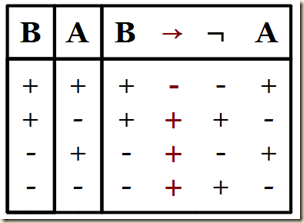So using this other terminology, we see that the case which ends up denying the whole conditional is the one where we both affirm B and affirm A. So look at that first row under the formula on the right-side box of the truth-table, and look specifically under B and under the negation symbol ¬ beside A. We see that row one is the only place where we both affirm B and deny the negation of A. Hence, as Frege wrote: “the case in which B is to be affirmed and the negation of A is to be denied does not occur”. We also see (as noted before) that in this line, both A and B are affirmed. Thus as Frege writes: “the possibility of affirming both A and B does not exist”. I am not sure how to explain the third formulation: “A and B are mutually exclusive”. We see that the whole conditional is affirmed when either term is affirmed. But the conditional is also affirmed when both terms are denied. I suppose that also is consistent with the idea of A and B being mutually exclusive. Frege continues:]

Thus only the three following cases remain:

A affirmed, B denied;
A denied, B affirmed;
A denied, B denied.
(8)

[As we can see from the truth table, these other three options are found in rows 2-4.]

We now consider: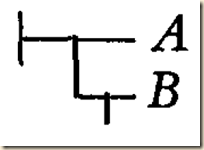This means:

‘the case in which A is denied and the negation of B is p.11] affirmed does not exist’; or, ‘A and B cannot both be denied.’ There remains only the following possibilities:

A affirmed, B affirmed;
A affirmed, B denied;
A denied, B affirmed.
(8)

[So let us look at a truth-table for the way we are construing Frege’s system.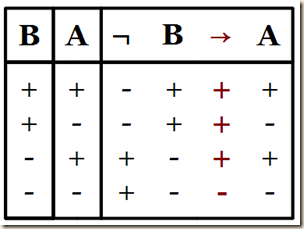Frege said this meant, “the case in which A is denied and the negation of B is affirmed does not exist”. We see in the fourth row that A is denied, the negation of B is affirmed, and the whole conditional is denied. Frege also wrote: “A and B cannot both be denied”. We see in the truth assignments on the left for the fourth row that both A and B are denied, but in that case, the whole conditional is denied. We also see that the three remaining possibilities Frege lists can be found in rows 1-3 of the table.]

Frege then says that there are two ways that we use “or” and “either-or”. It’ first meaning, “A or B” means the same as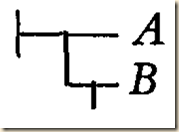or, “that nothing besides A and B is thinkable” (9a). [This would seem to be the inclusive disjunction.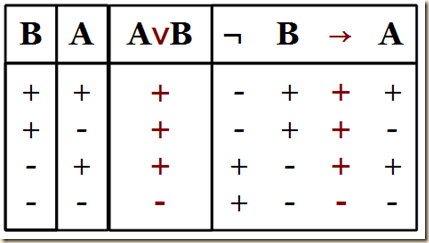] Frege gives an example: “if a gaseous mass is heated, then either its volume or its pressure increases” (9). [I am guessing this allows for both to increase as well.] [The second use seems to be the exclusive disjunction. He will say it combines the meanings of “B implies not-A” and “not-B implies A”. Perhaps he means something like the conjunction of the two. Consider this truth table. We see that this conjunction gives us the exclusive or evaluation.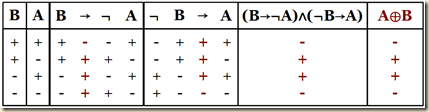] Frege writes:

Secondly the expression

A or B

may combine the meaning of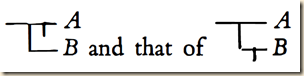so that (i) there is no third possibility besides A and B, (ii) A and B are mutually exclusive. In that case only the following two possibilities remain out of the four:

A affirmed, B denied;
A denied, B affirmed.
(9)

[Those two possibilities can be seen in rows 2 and 3 in the truth-table above.] Frege says that the first meaning of “or” is more important [but he does not explain why], and when we use “or” we will mean this sort of inclusive disjunction. If we had to distinguish “or” from “either-or”, then we should assign “either-or” the meaning of the exclusive disjunction (9).

[For the next meaning, we recall this distinction.

] Frege writes: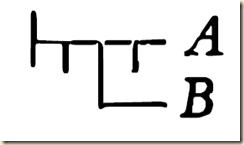means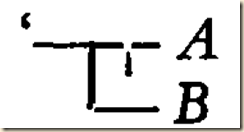is denied’ or ‘the case in which A and B are both affirmed occurs.’
(9)

[As we can see from the truth table, it is only in row one, where A and B are both affirmed, that the whole conditional is true.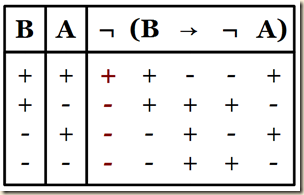] And thus, the three possibilities [rows 2-4] are excluded here, although they were the only ones included when this conditional was not negated [see the column under the conditional sign above] (9). And again, because it affirms both A and B, we can translate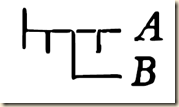“as both A and B are facts” (9d). [In other words, we can describe the conjunctive operator “and” using this formulation.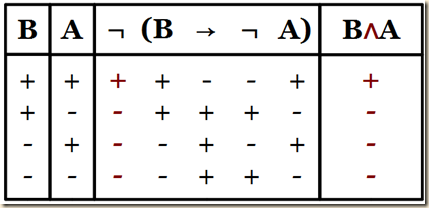]

So we have just [above] rendered “and” using the symbols for the conditional and for negation. We also could render the conditional using conjunction and negation. [In what follows, I think he is showing the following. The equivalent of BA is ¬(B∧¬A). We can see that with a truth table.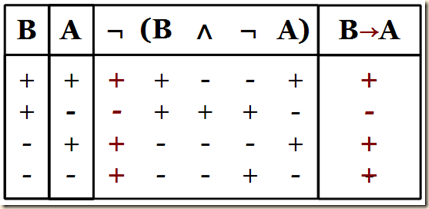He will propose a new symbol for conjunction. It will be like a left bracket, and whatever is stacked up on the right side will be the conjoined terms. He will use a little ‘T’ formation for negation. When it is placed next to a term or to the bracket conjoining terms, it means negation, I think. Let me quote:]

We might, say, introduce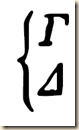as a symbol for the combined content of Γ and Δ and then
render p. 13]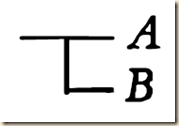by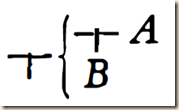I chose the other way because inference seemed to be expressed more simply that way.
(10)

Frege continues that we do not need to distinguish the meanings of “and” and “but” (10).

[Frege now will express the meaning for “neither ... nor.” Recall from section 2.5 of Agler’s Symbolic Logic that we translate “Neither P nor Q” as ¬P∧¬Q. Frege will render it using the conditional as: ¬(¬AB). As we can see, they are equivalent.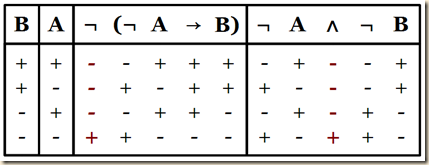Now quoting from Frege:]

means: ‘the case where A and B are both denied occurs.’ We may thus render it as:

‘neither A nor B is a fact.’

Clearly we are here concerned with the words ‘or,’ ‘and,’ ‘neither ... nor’ only in so far as they combine possible contents of judgment.
(10)

Frege, Gottlob. “Begriffsschrift (Chapter 1)”. Transl. P.T. Geach. In Translations from the Philosophical Writings of Gottlob Frege. Eds. P.T. Geach and Max Black. Oxford: Basil Blackwell, 1960, second edition (1952 first edition).

.

.